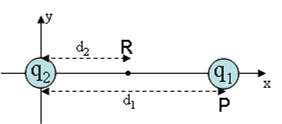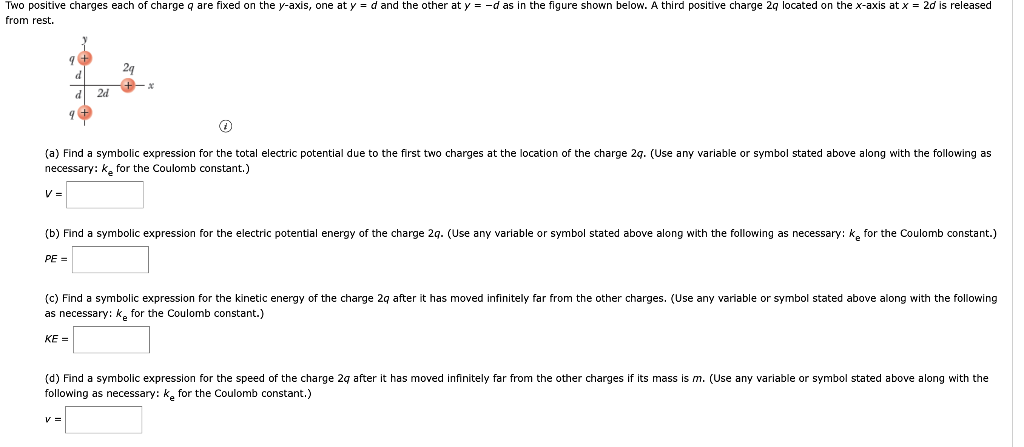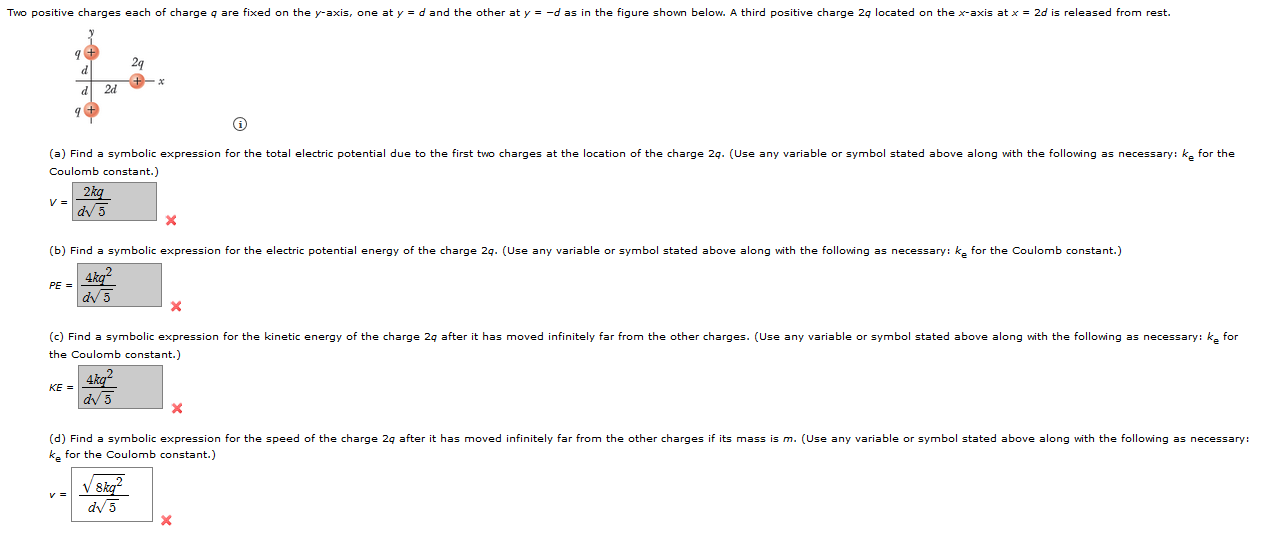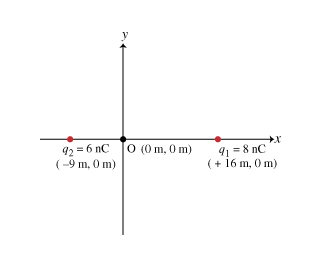Question

Distance between charges

Two charges are located along the x-axis. One has a charge of 6.2 μC, and the second has a charge of -3/5 μC. If the electrical potential energy associated withthe pair of charges is -0.05 J, what is the distance between the charges?

The Coulomb constant is 8.98756 x 109

We need at least 10 more requests to produce the answer.

0 / 10 have requested this problem solution

The more requests, the faster the answer.

All students who have requested the answer will be notified once they are available.

Earn Coins

Coins can be redeemed for fabulous gifts.

Similar Homework Help Questions
• Electric field potential energy problem. Physics 2. Will rate all and LS!

Two charges are located along the x-axis. One has a charge of 6.4 µC, and the second has a charge of -3.3 µC. The Coulomb constant is ke = 8.98755 × 109 Nm^2/C^2. Theacceleration of gravity is 9.81 m/s^2 . If the electrical potential energy associated with the pair of charges is -4.9×10-2 J, what is the distance between thecharges?Answer in units of m.

• A point charge q2 = -4.5 μC is fixed at the origin of a co-ordinate system...A point charge q2 = -4.5 μC is fixed at the origin of a co-ordinate system as shown. Another point charge q1 = 3.5 μC is is initially located at point P, a distance d1 = 7.2 cm from the origin along the x-axis 1) What is ΔPE, the change in potenial energy of charge q1 when it is moved from point P to point R, located a distance d2 = 2.9 cm from the origin along the x-axis as...

• Electric charges

Two positive charges each of charge q are fixed on the y-axis, one at y = d and the other at y = -d as in the figure shown below. A third positive charge 2q located onthe x-axis at x = 2d is released from rest.(a) Find a symbolic expression for the total electric potential due to the first two charges at the location of the charge 2q. (Use any variable or symbol stated abovealong with the following as necessary:...

• A 28-μC (1 μC = 10-6 coulomb) point charge is sitting at the origin. A 1.0-C...

A 28-μC (1 μC = 10-6 coulomb) point charge is sitting at the origin. A 1.0-C point charge is placed at (9.4 m, 0 m) on the x-axis. The electric potential energy between the two charges is (k = 8.99×109 Nm2/C2) Question 8 options: a) 27,000 J. b) 29,000 J. c) 2,500 J/C. d) b. 28,000 J.

• #1 and #2 are correct; I'm able to solve everything except #3 A point charge q2...#1 and #2 are correct; I'm able to solve everything except #3 A point charge q2 = -1.6 μC is fixed at the origin of a co-ordinate system as shown. Another point charge q1 = 1.9 μC is is initially located at point P, a distance d1 = 7.1 cm from the origin along the x-axis 1) What is ΔPE, the change in potenial energy of charge q1 when it is moved from point P to point R, located a...

• Two positive charges each of charge q are fixed on the y-axis, one at y =...Two positive charges each of charge q are fixed on the y-axis, one at y = d and the other at y = -d as in the figure shown below. A third positive charge 29 located on the x-axis at x = 2d is released from rest. a + 24 (a) Find a symbolic expression for the total electric potential due to the first two charges at the location of the charge 2g. (Use any variable or symbol stated above...

• Two positive charges each of charge q are fixed on the y-axis, one at y =...Two positive charges each of charge q are fixed on the y-axis, one at y = d and the other at y = -d as in the figure shown below. A third positive charge 27 located on the x-axis at x = 2d is released from rest. 9 + 29 d 24 +-* (a) Find a symbolic expression for the total electric potential due to the first two charges at the location of the charge 2q. (Use any variable or...

• Two point charges are placed on the x axis. Figure 1. The first charge, q1= 8.00nC,...Two point charges are placed on the x axis. Figure 1. The first charge, q1= 8.00nC, is placed a distance 16.0 m from the origin along the positive x axis; the second charge, q2= 6.00nC, is placed a distance 9.00 m from the origin along the negative x axis. a) find the electric field at the origin, point O.    Give the x and y components of the electric field as an ordered pair. Express your answer in newtons per coulomb....

• 1- How far from a -7.80 μC point charge must a 2.10 μC point charge be...

1- How far from a -7.80 μC point charge must a 2.10 μC point charge be placed in order for the electric potential energy of the pair of charges to be -0.500 J ? (Take the energy to be zero when the charges are infinitely far apart.) d= ------m 2- Two stationary positive point charges, charge 1 of magnitude 3.50 nC and charge 2 of magnitude 2.00 nC , are separated by a distance of 37.0 cm . An electron...

• A point charge 4.60 μC is held fixed at the origin. A second point charge 1.20...

A point charge 4.60 μC is held fixed at the origin. A second point charge 1.20 μC with mass of 2.80×10−4 kg is placed on the x axis, 0.220 m from the origin. What is the electric potential energy U of the pair of charges? (Take U to be zero when the charges have infinite separation.) U U = 0.226   J   The second point charge is released from rest. What is its speed when its distance from the origin is...